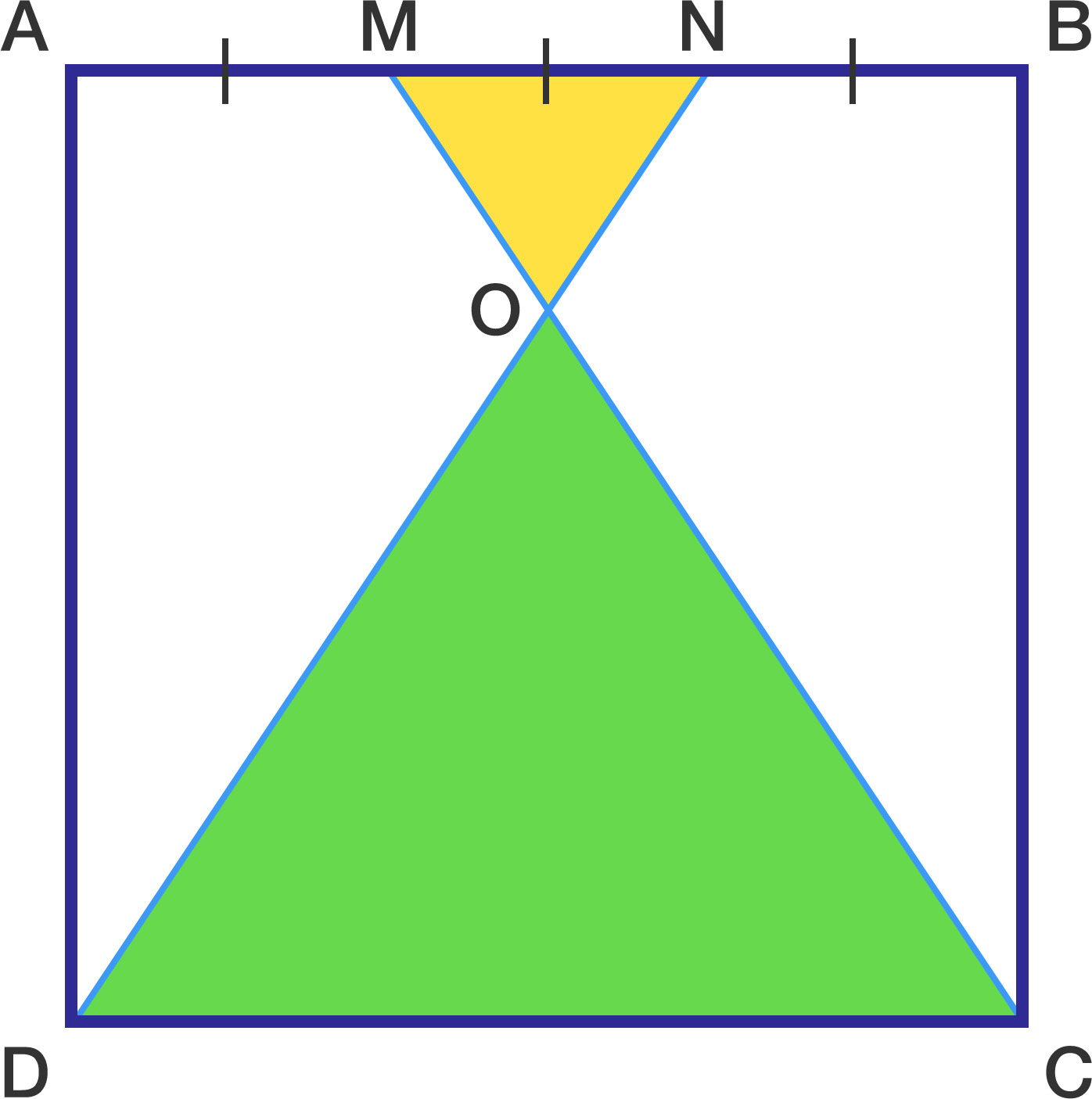# A geometry problem by Ajay Sambhriya

Geometry Level 2The above shows a square $ABCD$ of side length 12.

The points $M$ and $N$ are on the side length $AB$ such that $AM=MN = NB$.

Find the ratio of areas between the triangle $NOM$ and the triangle $DOC$.

×